Chapter 6 Part 1 - Foreign Exchange Rates

Economics Class 12
Macroeconomics

In this case, Exchange Rate of a currency is fixed by the Government

Exchange Rate does not fluctuate daily

(Example- Suppose Govt fix exchange Rate at Rs 70 per \$,it will remain the same unless govt changes it

This is done to ensure stability in foreign trade

Govt may Increase the exchange Rate to 80/\$ called Devaluation) or Decrease Exchange Rate to Rs 60 per \$ Called Revaluation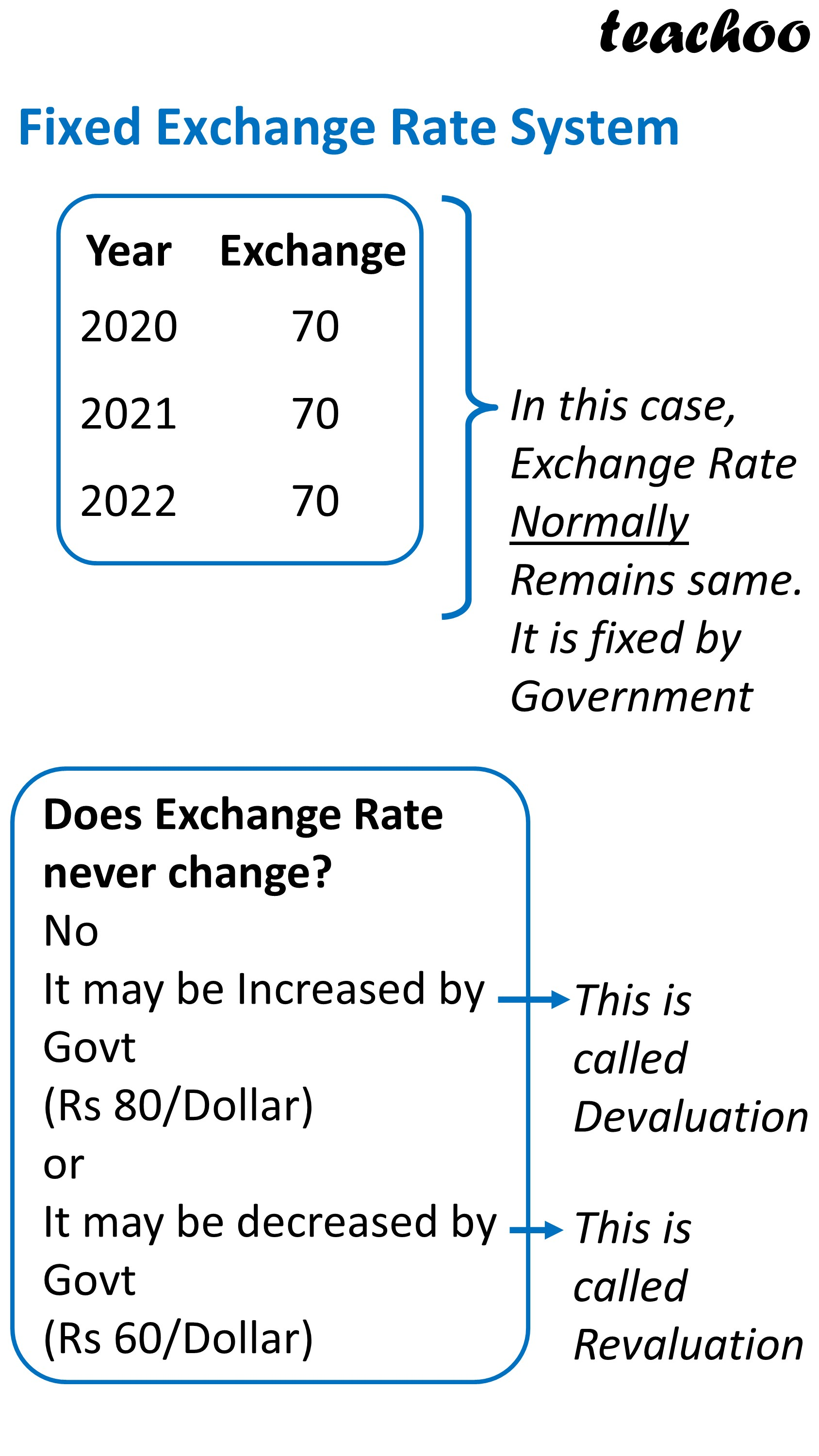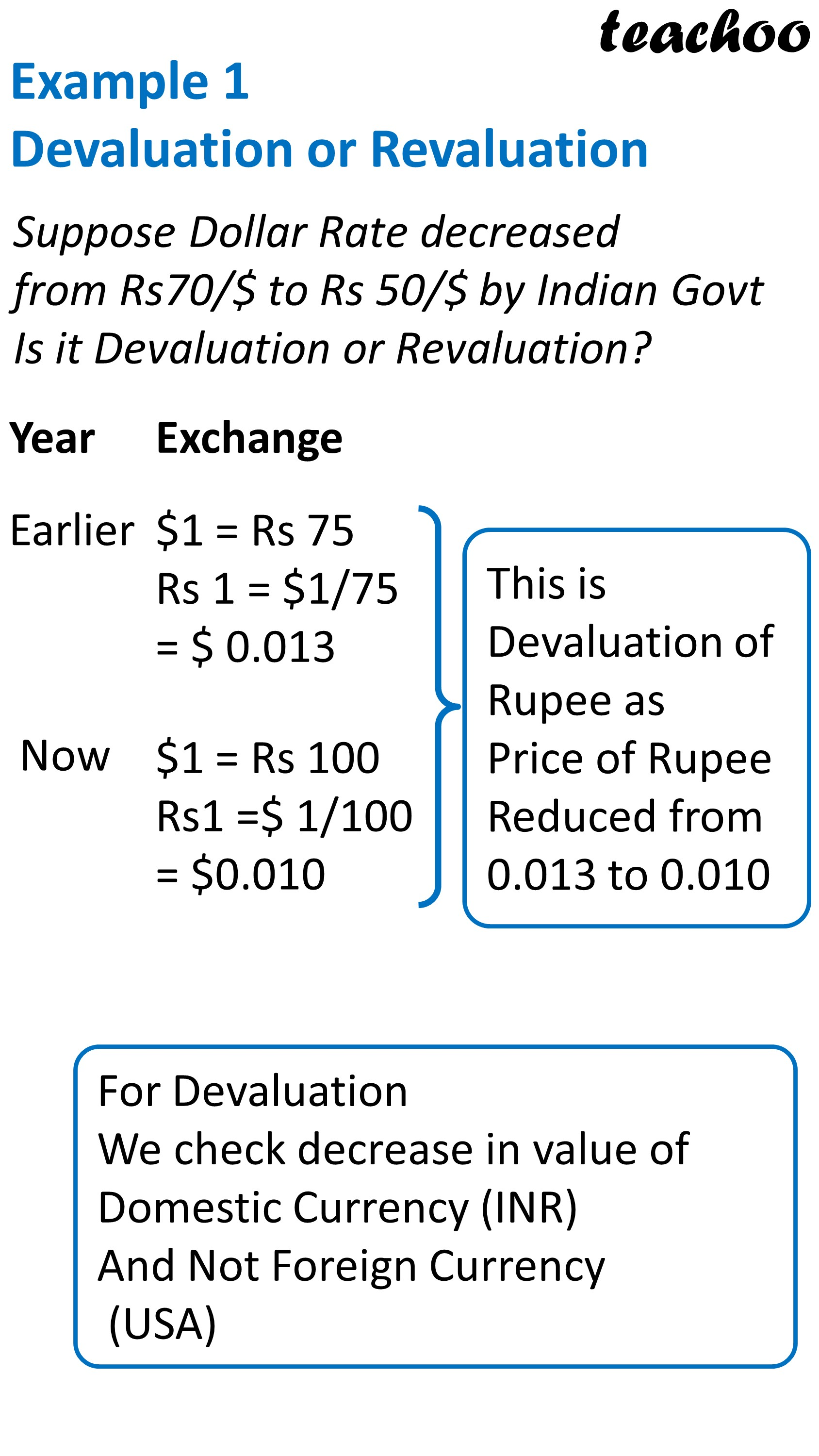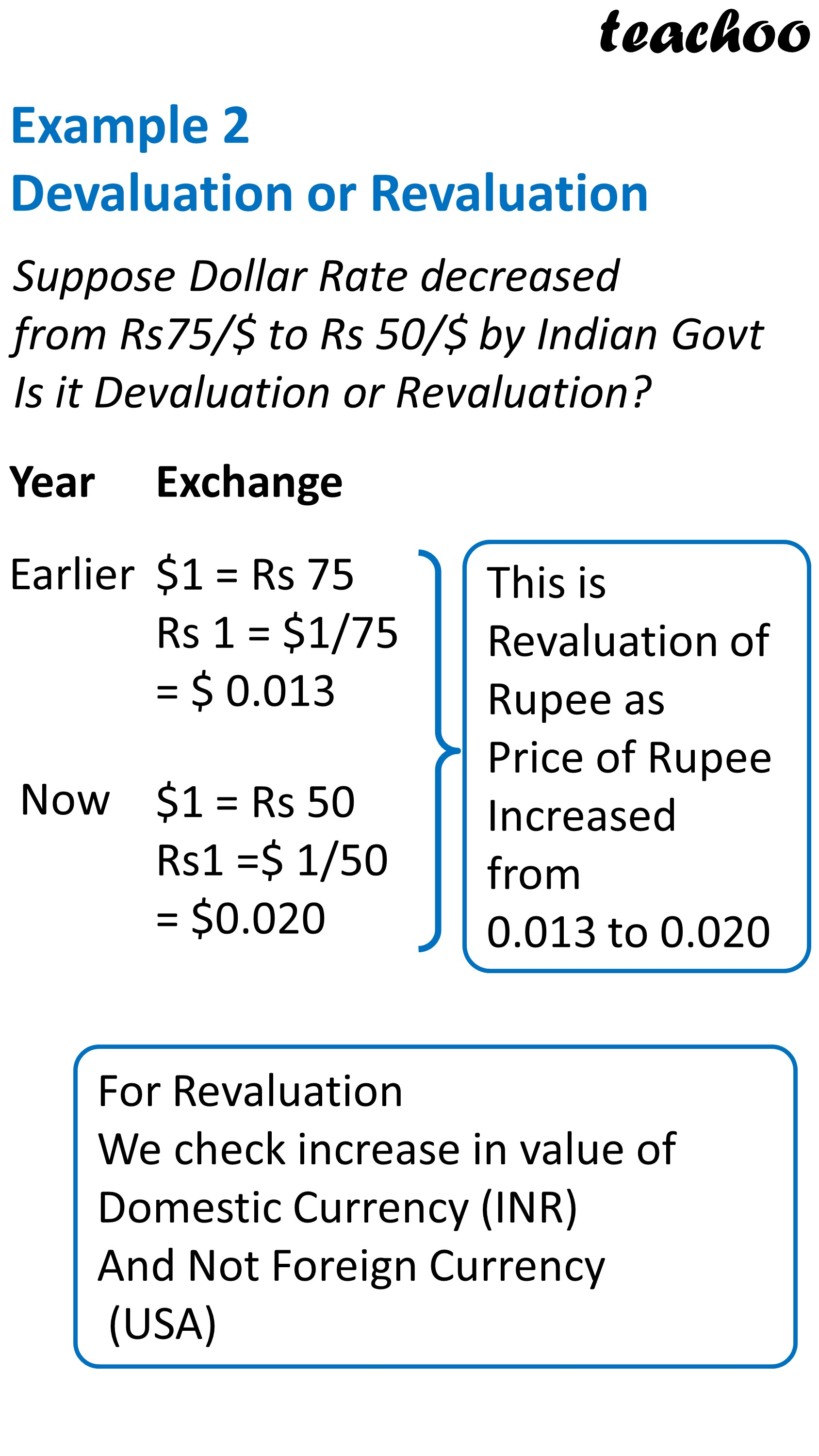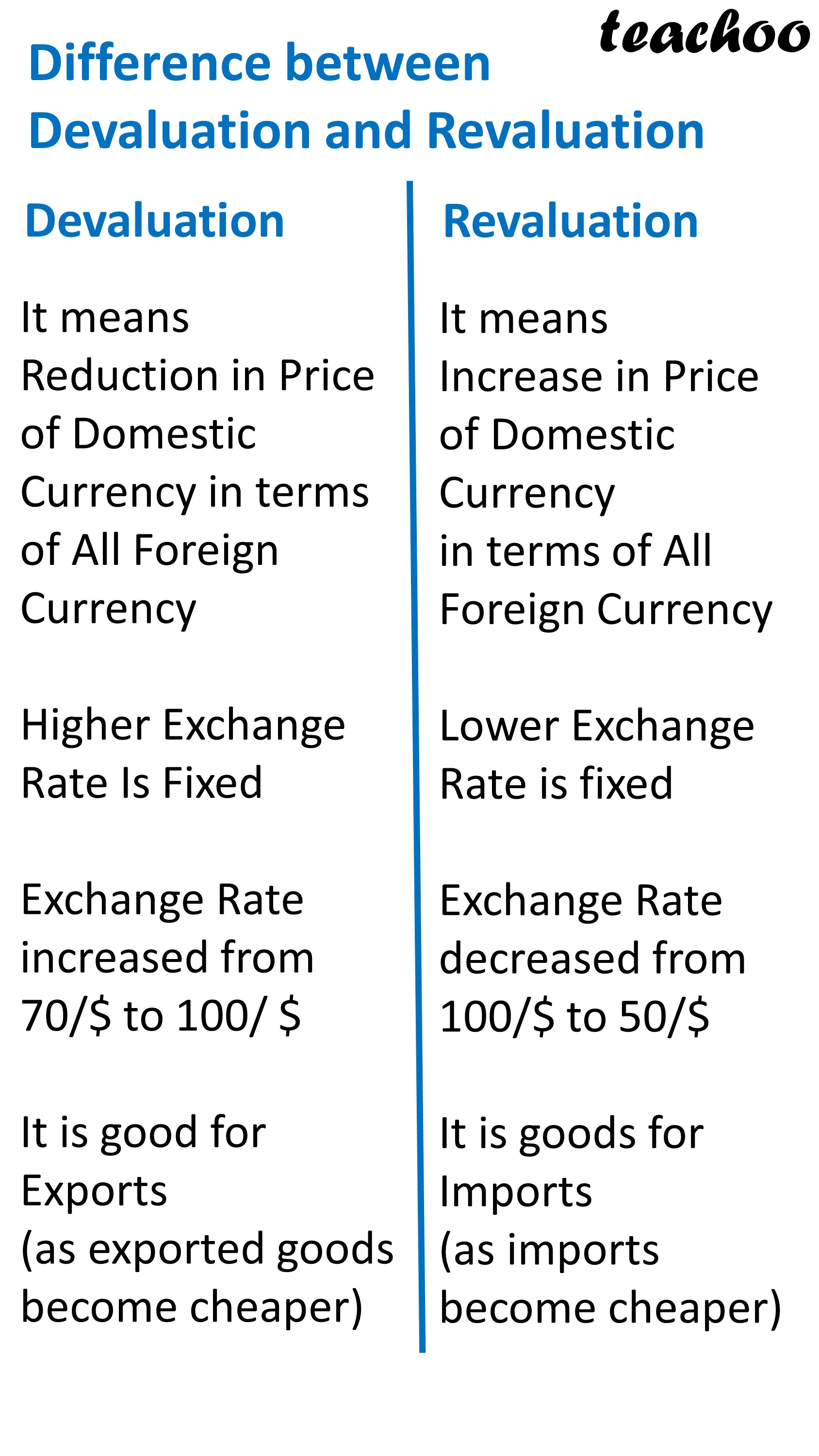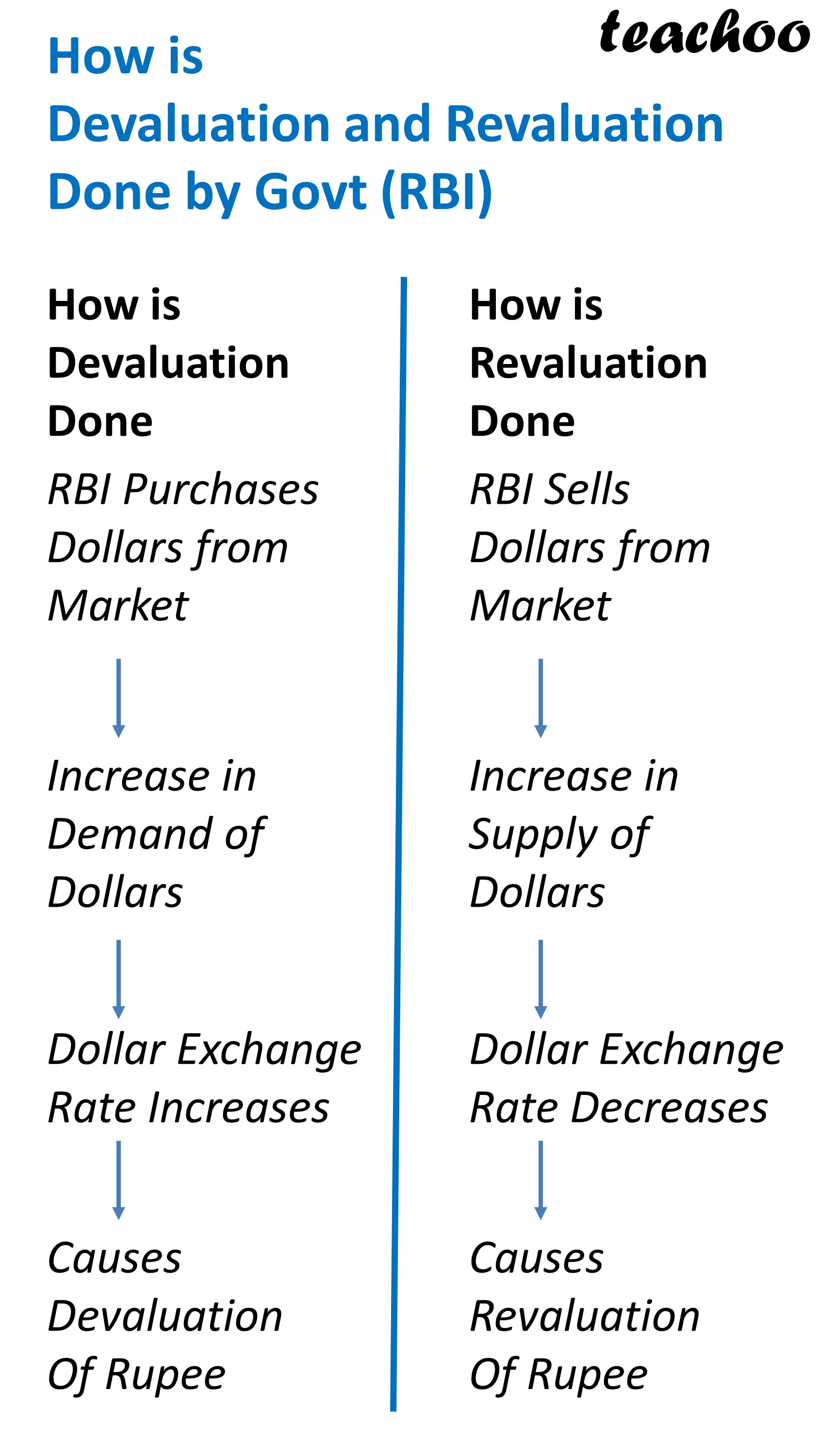## When is Devaluation Done

If Govt Wants to increase Exports

It will fix Higher Exchange Rate

Example - It will change Exchange Rate of Dollar from Rs 70 per \$ to Rs 80 per \$

Hence, it will make domestic currency cheaper as compared to foreign currency and in turn domestic goods cheaper

There will be excess supply of foreign currency in the market

To prevent this, Govt will Purchase Foreign Currency

## When is Revaluation done

In this case, Govt fixes Lower Exchange Rate

Example - It will change Exchange Rate of Dollar from Rs70 per \$ to Rs 60 per \$

Hence, it will increase value of domestic currency cheaper as compared to foreign currency

For doing this, Govt will Sell Foreign Currency

 Basis Devaluation Revaluation Meaning It means Reduction in Price of Domestic Currency in terms of all foreign currency It means Increase  in Price of Domestic Currency in terms of all foreign currency In this case, Higher Exchange Rate is fixed by Govt In this case, Lower Exchange Rate is fixed by the govt Example Exchange Rate increased from Rs 70 per \$ to Rs80 per \$ Exchange Rate decreased from Rs 70 per \$ to Rs60 per \$ Hence, Value of Rupee decreases as compared to dollar Hence, Value of Rupee increases as compared to dollar Method When the govt increases exchange rate When the govt decreases  exchange rate There is excess supply of foreign currency in market There is excess demand  of foreign currency in market So Govt buys foreign Exchange from the market Govt Sells foreign Exchange in the  Market

Summary

In fixed exchange Method

Govt keeps exchange rate fixed

Exchange Rate does not fluctuate daily

This is done to ensure stability in foreign trade

Govt may Increase Exchange Rate(Called Devaluation)

or

Govt may decrease Exchange Rate (Called Revaluation)

Difference between Fixed Exchange Rate and Flexible Exchange Rate

 Fixed Exchange Rate Flexible Exchange Rate In this case, Exchange Rate it  Fixed by Government In this case, Exchange Rate is determined by forces of demand and supply Exchange Rate Remains fixed and does not fluctuate Exchange Rate Fluctuates Daily There may be Devaluation or Revaluation of Currency by the Govt There may be Depreciation or Appreciation  of Currency due to change in demand and supply of foreign currency There is more stability of foreign trade It is less stable as exchange rate keeps on changing There is more role of Govt and Central Bank as it has to buy and sell foreign currency There is less role of govt as exchange rate is automatically determined This method is rarely used This method is normally used by all developed countries

## What is Pegging and Parity Value in Fixed Exchange Rate System?

### In Pegging

Exchange Rate of a currency is tied to some other currency or group of currency

Example

Saudi Arabia has pegged its currency Riyal to Dollar since 2003

Peg Rate is 3.75

It means

1 Riyal = 3.75 Dollar

### In Parity Value

Exchange Rate of a currency is fixed to an equal value of some commodity

Example

Price of gold in terms of rupee fixed at Rs 4800 /gram

Price of gold in terms of dolar fixed at \$ 60/gram

Hence, Equivalent Price of USA Dollar against rupee= 4800/60 = Rs 80/Dollar

### NCERT Questions

#### Question 7

Differentiate between devaluation and depreciation

#### Question 16

If inflation is higher in country A than in Country B, and the exchange rate between the two countries is fixed,

what is likely to happen to the trade balance between the two countries?

### Other Books

#### Question 1

In the following questions, select the correct answers:

Devaluation of currency means:

1. Reduction in the value of domestic currency by the market forces
2. Reduction in the value of domestic currency by the government
3. Both A and B
4. Neither A nor B

#### Question 2

When the government wants to strengthen the rupee, it ___ foreign currency and ___ domestic currency.

2. sells, sells

#### Question 3

The Gold Standard system includes:

1. Fixing values of foreign currencies IN terms of US Dollar
2. Fixing values of foreign currencies in terms of gold
3. Both A and B
4. None of these

### Oswaal Questions

#### Question 1

Which of the following statements is not true?

1. Depreciation of the foreign currency leads to the fall in exports.
2. Devaluation of the domestic currency leads to a rise in imports.
3. Appreciation of domestic currency leads to rise in exports.
4. Appreciation of foreign currency leads to fall in imports.

#### Question 2

Identify the correct pair as given in Column B by matching them with respective concepts in Column A:

 Column A Column B (1) Reduction in the value  of domestic currency by  the government (a) Devaluation (2) Reduction in the value  of domestic currency  through market forces (b) Appreciation (3) Increase in the value of  domestic currency by  the government (c) Depreciation (4) Increase in the value  of domestic currency  through market forces (d) Revaluation
1. 1 - (a)
2. 2 - (b)
3. 3 - (c)
4. 4 - (d)

#### Question 3

Assertion (A): When in order to buy 1 US dollar Rs 80 are needed instead of Rs 75, domestic currency shows depreciation.

Reason (R): Depreciation of domestic currency refers to fall in the value of domestic currency in terms of foreign currency caused by rise in foreign exchange rate in the foreign exchange market.

Mark the correct choice:

1. Both Assertion (A) and Reason (R) are true, and Reason (R) is the correct explanation of the Assertion (A).
2. Both Assertion (A) and Reason (R) are true, but Reason (R) is not the correct explanation of the Assertion (A).
3. Assertion (A) is true, but Reason (R) is false.
4. Assertion (A) is false, but Reason (R) is true.

Get live Maths 1-on-1 Classs - Class 6 to 12

### Transcript

Fixed Exchange Rate System Year 2020 2021 2022 Exchange 70 70 70 In this case, Exchange Rate Normally Remains same. It is fixed by Government Does Exchange Rate never change? No It may be Increased by Govt (Rs 80/Dollar) or It may be decreased by Govt (Rs 60/Dollar) This is called Devaluation This is called Revaluation Example 1 Devaluation or Revaluation Suppose Dollar Rate decreased from Rs70/\$ to Rs 50/\$ by Indian Govt Is it Devaluation or Revaluation? Year Exchange Earlier \$1 = Rs 75 Rs 1 = \$1/75 = \$ 0.013 Now \$1 = Rs 100 Rs1 =\$ 1/100 = \$0.010 This is Devaluation of Rupee as Price of Rupee Reduced from 0.013 to 0.010 For Devaluation We check decrease in value of Domestic Currency (INR) And Not Foreign Currency (USA) Example 2 Devaluation or Revaluation Suppose Dollar Rate decreased from Rs75/\$ to Rs 50/\$ by Indian Govt Is it Devaluation or Revaluation? Year Exchange Earlier \$1 = Rs 75 Rs 1 = \$1/75 = \$ 0.013 Now \$1 = Rs 50 Rs1 =\$ 1/50 = \$0.020 This is Revaluation of Rupee as Price of Rupee Increased from 0.013 to 0.020 For Revaluation We check increase in value of Domestic Currency (INR) And Not Foreign Currency (USA) Difference between Devaluation and Revaluation Devaluation Revaluation It means Reduction in Price of Domestic Currency in terms of All Foreign Currency Higher Exchange Rate Is Fixed Exchange Rate increased from 70/\$ to 100/ \$ It is good for Exports (as exported goods become cheaper) It means Increase in Price of Domestic Currency in terms of All Foreign Currency Lower Exchange Rate is fixed Exchange Rate decreased from 100/\$ to 50/\$ It is goods for Imports (as imports become cheaper)